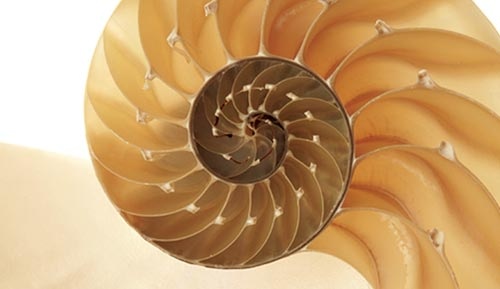# Calculus III

The third course in calculus, MAT 1236, continues the study of differential and integral calculus begun in MAT 1234 and 1235. The course includes parametric equations, polar coordinates, vectors, sequences, series, and Taylor expansions. It also introduces multivariable calculus, including partial derivatives, double integrals, and triple integrals.

The primary aims of the course are to help you develop new problem-solving and critical-reasoning skills and to prepare you for further study in mathematics, the physical sciences, or engineering. By the end of the course, you should be able to do the following:

• Sketch and analyze curves given parametrically.
• Graph curves in polar coordinates.
• Compute areas and arc lengths using rectangular and polar coordinates.
• Recognize and apply algebraic and geometric properties of vectors in two and three dimensions.
• Compute dot products and cross products and recognize their geometric meaning.
• Visualize and sketch surfaces in three-dimensional space.
• Compute and interpret partial derivatives of functions of several variables.
• Set up and evaluate double and triple integrals using a variety of coordinate systems, including rectangular, polar, cylindrical, and spherical.
• Determine the convergence of sequences and series by using standard tests.
• Determine the radius of convergence and interval of convergence of power series.
• Find the Taylor series of functions.
• Use Maple effectively to explore and solve calculus problems.
• Analyze and solve complex problems.
• Write short proofs using the ideas and techniques listed above.
• Provide clear written explanations of the ideas behind key concepts from the course.

You should also gain an increased appreciation of mathematics as part of the language of science and as a study in itself.#### Glimpsing God

“Thinking About the Infinite: A Mathematical Concept — and a Glimpse of God”: Insights from Dr. Robbin O’Leary